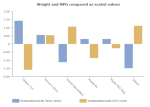# DCPY.STANDARDSCALER(with_mean, with_std, column)

The Standard Scaler scales the data by removing the mean and scaling it to the unit variance. It does not guarantee balanced feature scales in the presence of outliers as it is very sensitive to them.

###### Parameters
• with_mean – Specifies if the data needs to be centered by removing mean first, Boolean (for example, True).
• with_std – Specifies if the data needs to be scaled to unit variance, Boolean (for example, True).
• column – Dataset column or custom calculation that you want to scale.

Example: DCPY.STANDARDSCALER(True, True, [Discount])

###### Input data
• A numeric column
• Rows that contain missing values are dropped before calculations
###### Result
• A numeric column with transformed values with the same length as the input column
• Missing values are on the same indices like in the input column
###### Key usage points
• Use it when you need to normalize the data that does not contain a lot of outliers.
###### Example

The dataset values can vary in magnitudes, units, and range, but if we scale them both into comparable values, we can easily see how much one value is compared to the other. For example, we can scale the weight of the car and its fuel economy by using the following calculations: DCPY.STANDARDSCALER(True, True, [WT]) and DCPY.STANDARDSCALER(True, True, [MPG]).

Both calculations are visualized in the Butterfly chart,.For the whole list of algorithms, see Data science built-in algorithms.